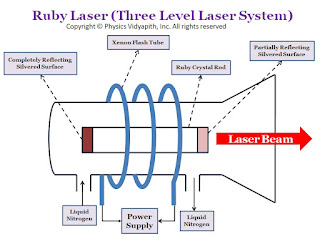Showing posts with label Control System. Show all posts
Showing posts with label Control System. Show all posts

## Mathematical modelling of control system

In order to design a control given application, the first and foremost  is to develop a mathematical  model of the system. Developing such a model implies that a definite mathematical relationship has to established between this system output and input to it. This approach provides and effective way of designing control systems.

The control systems are inherently dynamic in nature. Therefore the system dynamics are usually expressed as ordinary differential equations. Such an equation is called dynamic and governing equation of the system.

Control system is composed of real physical components fullestop these components may belong to electrical mechanical hydraulic or pneumatic domains. The dynamics of each class of these components is governed by certain well defined natural laws for example Kirchoff's law for electric components it and Newton's law and D Lambert's principles for mechanical components. In order to develop a mathematical model for control system, the natural laws governing the dynamics of its components are applied. Then from these equations are clear relationship between the system input and output can be established.

A control system may be modelled and analysed in frequency domain or in time domain fullestop transfer function is an example of mathematical model in frequency domain. On the other hand,a state space model is an example of modelling in time domain.

### Principle, Construction and Working of the Ruby Laser